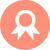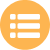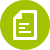## Servlet## WS## Hibernate# Java中HashMap之put方法的简介说明

## HashMap之put方法的简介

```HashMap之put方法
首先计算出key的hash值
然后检测出key是否存在，并进行相应的处理
```

## put源码

```public V put(K key, V value) {
return putVal(hash(key), key, value, false, true);
}

static final int hash(Object key) {
int h;
return (key == null) ? 0 : (h = key.hashCode()) ^ (h >>> 16);
}

//实现Map.put和相关方法
final V putVal(int hash, K key, V value, boolean onlyIfAbsent,
boolean evict) {
Node<K,V>[] tab; Node<K,V> p; int n, i;
// 步骤①：tab为空则创建
// table未初始化或者长度为0，进行扩容
if ((tab = table) == null || (n = tab.length) == 0)
n = (tab = resize()).length;
// 步骤②：计算index，并对null做处理
// (n - 1) & hash 确定元素存放在哪个桶中，桶为空，新生成结点放入桶中(此时，这个结点是放在数组中)
if ((p = tab[i = (n - 1) & hash]) == null)
tab[i] = newNode(hash, key, value, null);
// 桶中已经存在元素
else {
Node<K,V> e; K k;
// 步骤③：节点key存在，直接覆盖value
// 比较桶中第一个元素(数组中的结点)的hash值相等，key相等
if (p.hash == hash &&
((k = p.key) == key || (key != null && key.equals(k))))
// 将第一个元素赋值给e，用e来记录
e = p;
// 步骤④：判断该链为红黑树
// hash值不相等，即key不相等；为红黑树结点
// 如果当前元素类型为TreeNode，表示为红黑树，putTreeVal返回待存放的node, e可能为null
else if (p instanceof TreeNode)
// 放入树中
e = ((TreeNode<K,V>)p).putTreeVal(this, tab, hash, key, value);
// 步骤⑤：该链为链表
// 为链表结点
else {
// 在链表最末插入结点
for (int binCount = 0; ; ++binCount) {
// 到达链表的尾部

//判断该链表尾部指针是不是空的
if ((e = p.next) == null) {
// 在尾部插入新结点
p.next = newNode(hash, key, value, null);
//判断链表的长度是否达到转化红黑树的临界值，临界值为8
if (binCount >= TREEIFY_THRESHOLD - 1) // -1 for 1st
//链表结构转树形结构
treeifyBin(tab, hash);
// 跳出循环
break;
}
// 判断链表中结点的key值与插入的元素的key值是否相等
if (e.hash == hash &&
((k = e.key) == key || (key != null && key.equals(k))))
// 相等，跳出循环
break;
// 用于遍历桶中的链表，与前面的e = p.next组合，可以遍历链表
p = e;
}
}
//判断当前的key已经存在的情况下，再来一个相同的hash值、key值时，返回新来的value这个值
if (e != null) {
// 记录e的value
V oldValue = e.value;
// onlyIfAbsent为false或者旧值为null
if (!onlyIfAbsent || oldValue == null)
//用新值替换旧值
e.value = value;
// 访问后回调
afterNodeAccess(e);
// 返回旧值
return oldValue;
}
}
// 结构性修改
++modCount;
// 步骤⑥：超过最大容量就扩容
// 实际大小大于阈值则扩容
if (++size > threshold)
resize();
// 插入后回调
afterNodeInsertion(evict);
return null;
}
```
```①.判断键值对数组table[i]是否为空或为null，否则执行resize()进行扩容；

②.根据键值key计算hash值得到插入的数组索引i，如果table[i]==null，直接新建节点添加，转向⑥，如果table[i]不为空，转向③；

③.判断table[i]的首个元素是否和key一样，如果相同直接覆盖value，否则转向④，这里的相同指的是hashCode以及equals；

④.判断table[i] 是否为treeNode，即table[i] 是否是红黑树，如果是红黑树，则直接在树中插入键值对，否则转向⑤；

⑤.遍历table[i]，判断链表长度是否大于8，大于8的话把链表转换为红黑树，在红黑树中执行插入操作，否则进行链表的插入操作；遍历过程中若发现key已经存在直接覆盖value即可；

⑥.插入成功后，判断实际存在的键值对数量size是否超多了最大容量threshold，如果超过，进行扩容
```

#### 好文推荐

Java265.com

https://www.java265.com

### 站长统计|粤ICP备14097017号-3